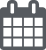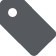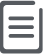# JavaScript正则表达式基础2019-01-07JavaScript387

ECMAScript 3 开始支持正则表达式，其语法和 Perl 语法很类似，一个完整的正则表达式结构如下：

``var expression = / pattern / flags ;``

g： 表示全局（global）模式，即模式将被应用于所有字符串，而非在发现第一个匹配项时立即停止；

i ： 表示不区分大小写（case-insensitive）模式，即在确定匹配项时忽略模式与字符串的大小写；

m：表示多行（multiline）模式，即在到达一行文本末尾时还会继续查找下一行中是否存在与模式匹配的项。

``````// 使用直接字面量创建
var exp1 = /(^\\s+)|(\\s+\$)/g;
// 使用RegExp对象创建
var exp2 = new RegExp('(^\\\\s+)|(\\\\s+\$)', 'g');  ``````

exp1 和 exp2 是两个完全等价的正则表达式，需要注意的是，传递给 RegExp 构造函数的两个参数都是字符串，不能把正则表达式字面量传递给 RegExp 构造函数。

``( \[ { \\ ^ \$ | ) ? \* + .\] }``

``````// 匹配 .docx
var exp = /\\.docx/gi ;``````

``````// 对 \\. 再次转义
var exp = new RegExp('\\\\.docx', 'gi');
//匹配 \\n
var exp1 = /\\\\n/g; //对\\n中的\\转义
var exp2 = new RegExp('\\\\\\\\n', 'g'); // 对 \\\\n 再次转义``````

### () [] {} 的区别

() 的作用是提取匹配的字符串。表达式中有几个()就会得到几个相应的匹配字符串。比如 (\s+) 表示连续空格的字符串。

[] 是定义匹配的字符范围。比如 [a-zA-Z0-9] 表示字符文本要匹配英文字符和数字。

{} 一般用来表示匹配的长度，比如 \d{3} 表示匹配三个数字，\d{1,3} 表示匹配1~3个数字，\d{3,} 表示匹配3个以上数字。

### ^ 与 \$

^ 匹配一个字符串的开头，比如 (^a) 就是匹配以字母a开头的字符串

\$ 匹配一个字符串的结尾,比如 (b\$) 就是匹配以字母b结尾的字符串

^ 还有另个一个作用就是取反，比如[^xyz] 表示匹配的字符串不包含xyz

^ 和 \$ 配合可以有效匹配完整字符串:

``````/d+/.test('4xpt');  // true - 部分匹配成功
/^\\d+\$/.test('4xpt');  // false - 完整匹配失败``````

### \d \s \w .

\d 匹配一个非负整数， 等价于 [0-9]

\s 匹配一个空白字符

\w 匹配一个英文字母或数字，等价于[0-9a-zA-Z]

. 匹配除换行符以外的任意字符，等价于[^\n]

### * + ?

* 表示匹配前面元素0次或多次，比如 (\s*) 就是匹配0个或多个空格

+ 表示匹配前面元素1次或多次，比如 (\d+) 就是匹配由至少1个整数组成的字符串

? 表示匹配前面元素0次或1次，相当于{0,1} ，比如(\w?) 就是匹配最多由1个字母或数字组成的字符串

### \$1 与 \1

\$1-\$9 存放着正则表达式中最近的9个正则表达式的提取的结果，这些结果按照子匹配的出现顺序依次排列。基本语法是：RegExp.\$n ，这些属性是静态的，除了replace中的第二个参数可以省略 RegExp 之外，其他地方使用都要加上 RegExp 。

``````//使用RegExp访问
/(\\d+)-(\\d+)-(\\d+)/.test('2016-03-26')
RegExp.\$1  // 2016
RegExp.\$2  // 03
RegExp.\$3  // 26
//在replace中使用
'2016-03-26'.replace(/(\\d+)-(\\d+)-(\\d+)/, '\$1年\$2月\$3日')
// 2016年03月26日``````

\1 表示后向引用，是指在正则表达式中，从左往右数，第1个()中的内容，以此类推，\2表示第2个()，\0表示整个表达式。

``````//匹配日期格式，表达式中的\\1代表重复(\\-|\\/|.)
var rgx = /\\d{4}(\\-|\\/|.)\\d{1,2}\\1\\d{1,2}/
rgx.test('2016-03-26') // true
rgx.test('2016-03.26') // false``````

### test 与 match

``````/\\d+/.test('123') ; // true
/\\d+/.test('abc') ; // false``````

match是获取正则匹配到的结果，以数组的形式返回

``'186a619b28'.match(/\\d+/g); // \['186', '619', '28'\]``

### replace

replace 本身是JavaScript字符串对象的一个方法，它允许接收两个参数：

``replace(\[RegExp|String\], \[String|Function\])``

result: 本次匹配到的结果

\$1,...\$9: 正则表达式中有几个()，就会传递几个参数，\$1~\$9分别代表本次匹配中每个()提取的结果，最多9个

offset: 记录本次匹配的开始位置

source: 接受匹配的原始字符串

### 经典案例

【1】实现字符串的 trim 函数，去除字符串两边的空格。

``````String.prototype.trim = function () {
// 方式一：将匹配到的每一个结果都用''替换
return this.replace(/(^\\s+)|(\\s+\$)/g, function(){
return '';
});
// 方式二：和方式一的原理相同
return this.replace(/(^\\s+)|(\\s+\$)/g, '');
};``````

^\s+ 表示以空格开头的连续空白字符，\s+\$ 表示以空格结尾的连续空白字符，加上() 就是将匹配到的结果提取出来，由于是 | 的关系，因此这个表达式最多会match到两个结果集，然后执行两次替换：

``````String.prototype.trim = function () {
/\*\*
\* @param rs：匹配结果
\* @param \$1:第1个()提取结果
\* @param \$2:第2个()提取结果
\* @param offset:匹配开始位置
\* @param source：原始字符串
\*/
this.replace(/(^\\s+)|(\\s+\$)/g, function(rs, \$1, \$2, offset, source){
// arguments中的每个元素对应一个参数
console.log(arguments);
});
};
' abcd '.trim();

\[' ', ' ', undefined, 0, ' abcd '\] // 第1次匹配结果
\[' ', undefined, ' ', 5, ' abcd '\] // 第2次匹配结果``````

【2】提取浏览器 url 中的参数名和参数值，生成一个key/value 的对象。

``````function getUrlParamObj(){
var obj = {};
//获取url的参数部分
var params = window.location.search.substr(1);
//\[^&=\]+ 表示不含&或=的连续字符，加上()就是提取对应字符串
params.replace(/(\[^&=\]+)=(\[^&=\]\*)/gi, function(rs, \$1, \$2){
obj\[\$1\] =  decodeURIComponent(\$2);
});
return obj;
}``````

/([^&=]+)=([^&=]*)/gi 每次匹配到的都是一个完整key/value，形如 xxxx=xxx, 每当匹配到一个这样的结果时就执行回调，并传递匹配到的 key 和 value，对应到\$1和\$2 。

【3】扩展 typeof，包含引用类型的具体类型。

``````function getDataType(obj){
let rst = Object.prototype.toString.call(obj);
rst = rst.replace(/\\\[object\\s(\\w+)\\\]/,'\$1'); // \[object Xxx\]
return rst.toLowerCase()
}
getDataType(1); // number
getDataType('a'); // string
getDataType(null); // null
getDataType(\[\]); // array``````

\$1 是正则表达式中第一个() 中匹配的内容。

replace 的第二个参数只能是字符串或函数，这里的 \$1 需要放在引号中。

【4】在字符串指定位置插入新字符串。

``````String.prototype.insetAt = function(str, offset){
offset = offset + 1;
//使用RegExp()构造函数创建正则表达式
var regx = new RegExp("(^.{"+offset+"})");
return this.replace(regx, '\$1' + str);
};
'abcd'.insetAt('xyz',2); // 在c字符后插入xyz
> 'abcxyzd'``````

【5】将手机号 12988886666 转化成 129****6666 。

``````function telFormat(tel){
tel = String(tel);
// 方式一
return tel.replace(/(\\d{3})(\\d{4})(\\d{4})/, function (rs, \$1, \$2, \$3) {
return \$1 + '\*\*\*\*' + \$3
});
// 方式二
return tel.replace(/(\\d{3})(\\d{4})(\\d{4})/, '\$1\*\*\*\*\$3');
}``````

(\d{3}\d{4}\d{4}) 可以匹配完整的手机号，并分别提取前 3 位、4-7 位和 8-11位，"\$1****\$3" 是将第 2 个匹配结果用****代替并组成新的字符串，然后替换完整的手机号。

【6】实现HTML编码，将< / > " & ` 等字符进行转义，避免 XSS 攻击 。

``````function htmlEncode(str) {
//匹配< / > " & \`
return str.replace(/\[<>"&\\/\`\]/g, function(rs) {
switch (rs) {
case "<":
return "<";
case ">":
return ">";
case "&":
return "&";
case "\\"":
return """;
case "/":
return "/"
case "\`":
return "'"
}
});
}``````

PREVIOUS:
NEXT: